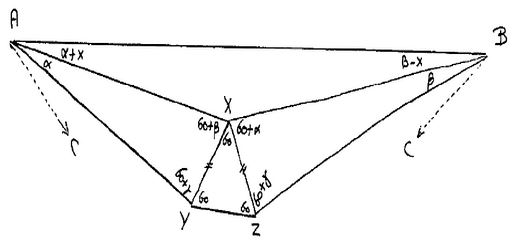# Morley's MiracleDijkstra's Proof

I lifted this proof from the site of E. W. Dijkstra Archives at the University of Texas at Austin.

An open letter to Ross Honsberger.
University of Waterloo.

30 December 1975

Dear Sir,

the other day I encountered your delightful booklet "Mathematical Gems". On account of Chapter 8, I concluded that you might be interested in the following proof of Morley's Theorem "The adjacent pairs of the trisectors of a triangle always meet at the vertices of an equilateral triangle."Choose $\alpha,$ $\beta$ & $\gamma \gt 0$ such that $\alpha + \beta + \gamma = 60^{\circ}.$ Draw an equilateral triangle $XYZ$ and construct the triangles $AXY$ and $BXZ$ with the angles as indicated. Because $\angle AXB = 180^{\circ} � (\alpha +\beta ),$ it follows that, if $\angle BAX = \alpha +x,$ $\angle ABX = \beta � x.$ Using the rule of sines three times (in $\Delta AXB,$ $\Delta AXY,$ and $\Delta BXZ),$ we deduce

\begin{align}\displaystyle \frac{\sin (\alpha +x)}{\sin (\beta � x)}&=\frac{BX}{AX}\\ &=\frac{XZ\cdot\sin (60^{\circ}+\gamma)/\sin (\beta )}{XY\cdot\sin (60^{\circ}+\gamma)/\sin (\alpha )}\\ &=\frac{\sin (\alpha )}{\sin (\beta )}. \end{align}

Because in the range considered, this equation has a left-hand-side which is a monotonically increasing function of $x$ (on account of the monotonicity of $\sin(\phi )$ in the first quadrant) we conclude $x = 0.$ Thus Morley�s Theorem is proved without any additional lines. I found this proof in the early sixties, but am afraid that I did not publish it. Yours ever,

Edsger W.Dijkstra### Morley's Miracle

#### Invalid proofs